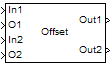# Offset

Truncate vectors by removing or keeping beginning or ending values

## Library

Signal Operations

`dspsigops`

•## Description

The Offset block removes or keeps values from the beginning or end of the input vectors. You specify the length of the output vectors using the Output port length parameter. The inputs to the In ports (In1, In2, ...) can be scalars or vectors, but they must be the same size and data type. The offset values are the inputs to the O ports (O1, O2, ...); they must be scalar values with the same data type. These offset values should be integer values because they determine the number of values the block discards or retains from each input vector. The block rounds any offset value that is a noninteger value to the nearest integer value. There is one output port for each pair of In and O ports.

Use the Mode parameter to determine which values the block discards or retains from the input vector. To discard the initial values of the vector, select `Remove beginning samples`. To discard the final values of the vector, select `Remove ending samples`. To retain the initial values of the vector, select `Keep beginning samples`. To retain the final values of a vector, select `Keep ending` samples.

Use the Number of input data-offset pairs parameter to specify the number of inputs to the block. The number of input ports is twice the scalar value you enter. For example, if you enter `3`, ports In1, O1, In2, O2, In3, and O3 appear on the block.

The block uses the Output port length parameter to determine the length of the output vectors. If you select `Same as input`, the block outputs vectors that are the same length as the input to the In ports. If you select `User-defined`, the Output length parameter appears. Enter a scalar that represents the desired length of the output vectors. If your desired output length is greater than the number of values you extracted from your input vector, the block zero-pads the end of the vector to reach the length you specified.

Use the Action for out of range offset value parameter to determine how the block behaves when an offset value is not in the range 0 ≤ offset value ≤ N, where N is the input vector length. Select `Clip` if you want any offset values less than 0 to be set to 0 and any offset values greater than N to be set to N. Select `Clip and warn` if you want to be warned when any offset values less than 0 are set to 0 and any offset values greater than N are set to N. Select `Error` if you want the simulation to stop and display an error when the offset values are out of range.

## Parameters

Mode

Use this parameter to determine which values the block discards or retains from the input vector. Your choices are ```Remove beginning samples```, `Remove ending samples`, `Keep beginning samples`, and ```Keep ending samples```.

Number of input data-offset pairs

Specify the number of inputs to the block. The number of input ports is twice the scalar value you enter.

Output port length

Use this parameter to specify the length of the output vectors. If you select `Same as input`, the output vectors are the same length as the input vectors. If you select `User-defined`, you can enter the desired length of the output vectors.

Output length

Enter a scalar that represents the desired length of the output vectors. This parameter is visible if, for the Output port length parameter, you select `User-defined`.

Action for out of range offset value

Use this parameter to determine how the block behaves when an offset value is not in the range such that 0 ≤ offset value ≤ N, where N is the input vector length. When you want any offset values less than 0 to be set to 0 and any offset values greater than N to be set to N, select `Clip`. When you want to be warned when any offset values less than 0 are set to 0 and any offset values greater than N are set to N, select `Clip and warn`. When you want the simulation to stop and display an error when the offset values are out of range, select `Error`.

## Supported Data Types

PortSupported Data Types

In

• Double-precision floating point

• Single-precision floating point

• Fixed point (signed only)

• 8-, 16-, and 32-bit signed integers

O

• Double-precision floating point

• Single-precision floating point

• 8-, 16-, and 32-bit signed integers

• 8-, 16-, and 32-bit unsigned integers

Out

• Double-precision floating point

• Single-precision floating point

• Fixed point (signed only)

• 8-, 16-, and 32-bit signed integers

## Extended Capabilities

Introduced before R2006a

## SupportGet trial now